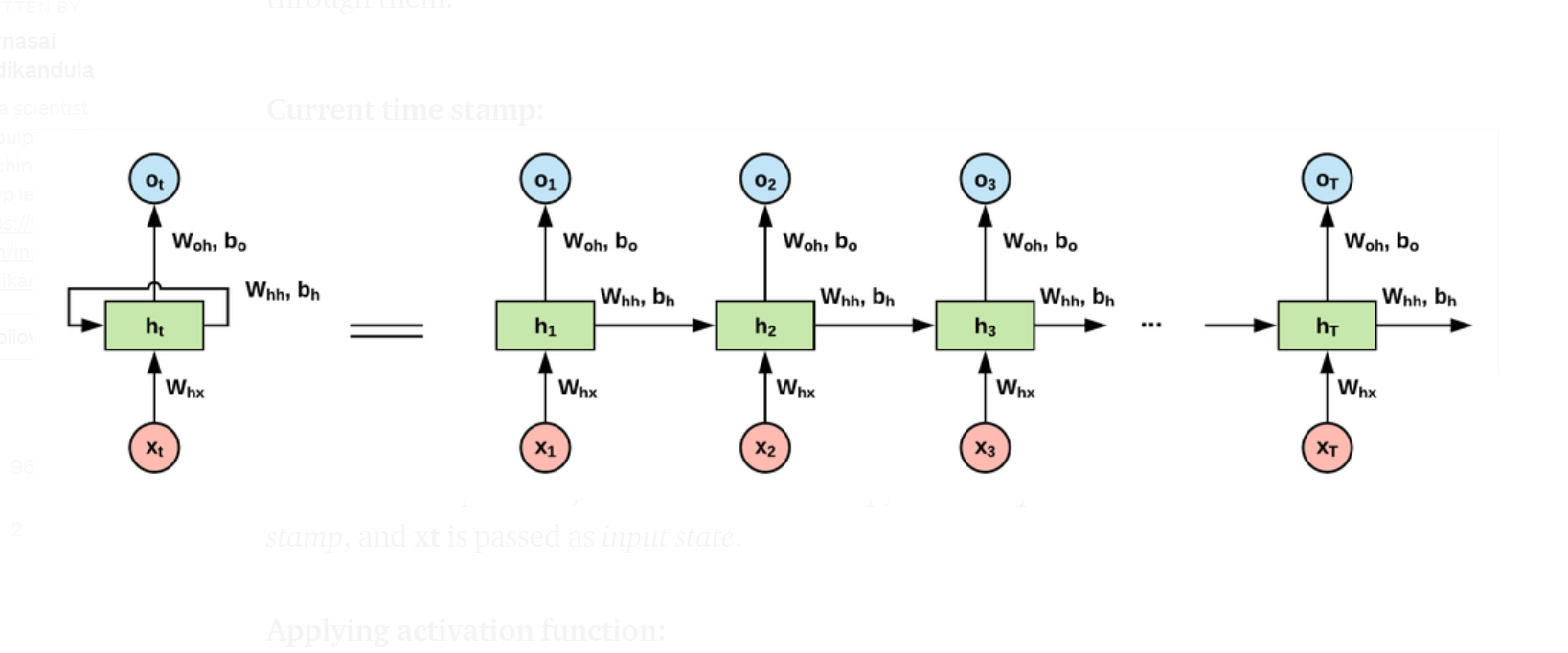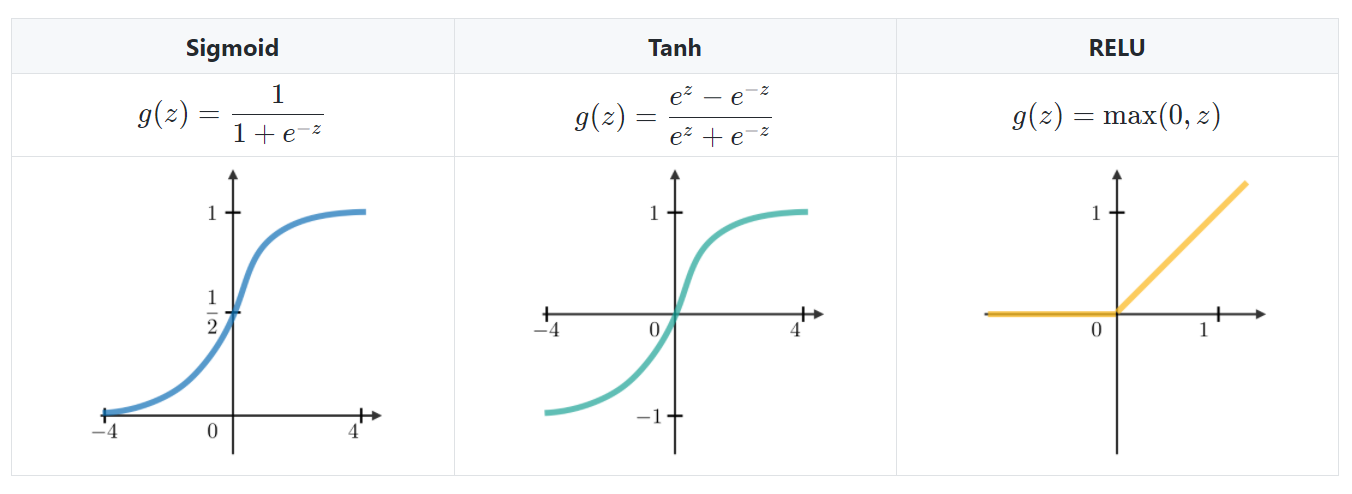×

Search anything:

#### Machine Learning (ML) rnnGet this book -> Problems on Array: For Interviews and Competitive Programming

Recurrent Neural Networks (or RNNs) are the first of their kind neural networks that can help in analyzing and learning sequences of data rather than just instance-based learning. Just like any other ML techniques it has some disadvantages which leaves the door open for improvement.

The main Disadvantages of RNNs are:

• Training RNNs
• The vanishing or exploding gradient problem
• RNNs cannot be stacked up
• Slow and Complex training procedures
• Difficult to process longer sequences

## Introduction

Before we get into the problems with RNNs, let us discuss why they were made in the first place? RNNs were created to learn from sequential data. What it means is that if you want the model to remember which instances came before the previous one, in order, then you need a recurrent model. For example, if you have a shadow to predict if it is a dog or a wolf, which one would you pick?

Side note: Wolf and dog, both of them mostly have the same outline (or shadow)

The thing is, to recognize that shadow in real life will require some context. This context can be learned from previous images given to the model. If the previous set contained trees, forests, and wild animals, we can very well say it must be a wolf. But if the previous set shows residential areas, the shadow must be a dog. But what if you want your model to do the same? It will need to remember previous inputs too. This is where RNNs come into the picture.

They are used extensively in applications like:

• Music generation
• Sentiment classification
• Name entity recognition
• Machine translation

Well, all of this looks great. What went wrong?

Although, RNNs are great at sequence data they are not perfect. To understand the exact problem, let us revise how RNNs are trained.

# Training RNNsThe above image shows quite nicely how a typical RNN block looks like. As you can see, RNNs take the previous node’s output as input in the current state too. Well, it does help in getting the context correct but fails in one aspect. Minimizing the loss function. Below are the typically used activation functions with RNNs.Source: Stanford.edu

Now, the problem with these activation functions is that whenever they are used in sequential training, the weights (or the gradients) make the process a bit tricky. Here is our first limitation.

# The vanishing or exploding gradient problem

The vanishing and/or exploding gradient problems are regularly experienced with regards to RNNs. The motivation behind why they happen is that it is hard to catch long haul conditions as a result of a multiplicative angle that can be dramatically diminishing/expanding regarding the number of layers. So, if at all the sequence gets too long, the model may train with null weights (i.e. no training) or exploding weights.

The training of any unfolded RNN is done through multiple time steps, where we calculate the error gradient as the sum of all gradient errors across timestamps. Hence the algorithm is also known as backpropagation through time (BPTT). Due to the chain rule application while calculating the error gradients, the domination of the multiplicative term increases over time and due to that the gradient has the tendency to explode or vanish.

If the largest eigenvalue is less than 1, the gradient will vanish. If the largest eigenvalue is more than 1, the gradient explodes.

The problem of exploding gradients can be solved using gradient clipping. As the name suggests, the gradients are clipped once they reach a pre-defined threshold. But the problem of vanishing gradients is still out there. It was later solved up to a point with the introduction of LSTM networks.

There are other methods for solving this problem of eigenvalue dependent gradient manipulation. For example, the L1 and L2 penalty of the recurrent weights and gradients. It is a simple hack/technique through which we can normalize or cut-off the eigenvalues when it goes to high in the multiplicative value.

# RNNs cannot be stacked up

The number one problem when it comes to parallelizing the trainings in RNN or a simple stack up of training is due to the fundamental characteristic of RNN, i.e., they are connected. What this means is RNNs require the output of the previous node to do the computation over the present node. Due to this connection, RNNs are not suitable for parallelizing or stacking up with other models. The overall computational expense that goes on can never be justified with any accuracy gain.

# Slow and Complex training procedures

One of the fundamental problems with RNNs is that they are recurrent. Meaning, they will take a lot of time for training. The overall training speed of RNN is quite low compared to feedforward networks. Secondly, as RNN needs to calibrate the previous outputs as well as current inputs into a state change function per node, it is quite difficult to implement. The complexity of training sometimes makes it tougher to customize RNN training.

# Difficult to process longer sequences

As discussed earlier, it is quite difficult to train RNNs on too long sequences, especially while using ReLU or tanh activations. This is another reason for introducing the GRU based networks.

# Conclusion

Overall, RNNs are quite useful and helps in making many things possible, from music to voice assistants. But the above problems are ones needed to be tackled. Solutions like LSTM networks and gradient clippings are now becoming an industry practice. But what if the core structure could be reformatted. Let's see what new additions are there in the RNN family.